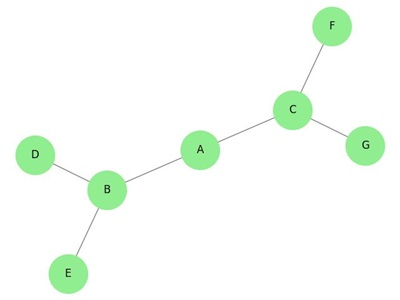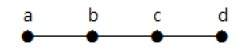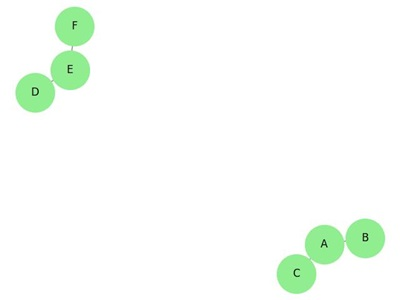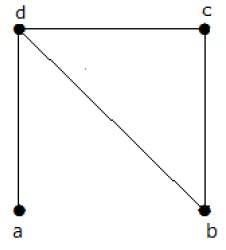# Tree or Connected acyclic graph

Trees are graphs that do not contain even a single cycle. They represent hierarchical structure in a graphical form. Trees belong to the simplest class of graphs. Despite their simplicity, they have a rich structure.

Trees provide a range of useful applications as simple as a family tree to as complex as trees in data structures of computer science.

## Tree

A connected acyclic graph is called a tree. In other words, a connected graph with no cycles is called a tree.

The edges of a tree are known as branches. Elements of trees are called their nodes. The nodes without child nodes are called leaf nodes.

A tree with 'n' vertices has 'n-1' edges. If it has one more edge extra than 'n-1', then the extra edge should obviously has to pair up with two vertices which leads to form a cycle. Then, it becomes a cyclic graph which is a violation for the tree graph.

### Example 1

The graph shown here is a tree because it has no cycles and it is connected. It has four vertices and three edges, i.e., for 'n' vertices 'n-1' edges as mentioned in the definition.Note − Every tree has at least two vertices of degree one.

### Example 2In the above example, the vertices 'a' and 'd' has degree one. And the other two vertices 'b' and 'c' has degree two. This is possible because for not forming a cycle, there should be at least two single edges anywhere in the graph. It is nothing but two edges with a degree of one.

## Forest

A disconnected acyclic graph is called a forest. In other words, a disjoint collection of trees is called a forest.

## Example

The following graph looks like two sub-graphs; but it is a single disconnected graph. There are no cycles in this graph. Hence, clearly it is a forest.## Spanning Trees

Let G be a connected graph, then the sub-graph H of G is called a spanning tree of G if −

• H is a tree
• H contains all vertices of G.

A spanning tree T of an undirected graph G is a subgraph that includes all of the vertices of G.

## ExampleIn the above example, G is a connected graph and H is a sub-graph of G.

Clearly, the graph H has no cycles, it is a tree with six edges which is one less than the total number of vertices. Hence H is the Spanning tree of G.

Updated on: 23-Aug-2019

1K+ Views